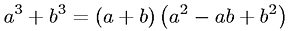Equations > Algebra > Polynomials > Sum of Cubes Factorization

### Sum of Cubes FactorizationLatex Code:

MathML Code:

 ${a}^{3}+{b}^{3}=\left(a+b\right)\left({a}^{2}-\mathrm{ab}+{b}^{2}\right)$

MathType 5.0: